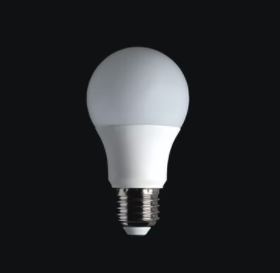## Motion Sensors to Reduce Costs

The lighting needs of a storage room are being met by 6 fluorescent light fixtures, each fixture containing four lamps rated at 60 W each. All the lamps are on during operating hours of the facility, which are 6 AM to 6 PM 365 days a year. The storage room is actually used for an average of 3 hours a day. If the price of electricity is $0.08/kWh, determine the amount of energy and money that will be saved as a result of installing motion sensors. Also, determine the simple pay-back period if the purchase price of the sensor is$32 and it takes 1 hour to install it at a cost of $40.Hint Energy Savings = (Number of lamps)(Lamp wattage)(Reduction of annual operating hours) Hint 2 Cost Savings = (Energy Savings)(Unit cost of energy) Assumptions : The electrical energy consumed by the ballasts is negligible. The plant operates 12 hours a day, and currently the lights are on for the entire 12 hour period. The motion sensors installed will keep the lights on for 3 hours, and off for the remaining 9 hours every day. This corresponds to a total of 9×365 = 3285 off hours per year. Disregarding the ballast factor, the annual energy and cost savings become Energy Savings = (Number of lamps)(Lamp wattage)(Reduction of annual operating hours) = (24 lamps)(60 W/lamp)(3285 hours/year) = 4730 kWh/year Cost Savings = (Energy Savings)(Unit cost of energy) = (4730 kWh/year)($0.08/kWh)
= $378/year The implementation cost of this measure is the sum of the purchase price of the sensor plus the labor. Implementation Cost = Material + Labor =$32 + $40 =$72
Solving for the payback period:
$$Payback\:Period=\frac{Implementation\:Cost}{Annual\:Cost\:Savings}$$$$$=\frac{72}{378}=0.19\:year\:(2.3\:months)$$$
Energy Savings = 4730 kWh/year
Cost Savings = \$378/year
Payback Period = 0.19 year (2.3 months) or ~2 months